Next: 4.3 Simulation in FEDOS Up: 4.2 Discretization of the Previous: 4.2.3 Discretization of the

## 4.2.4 Discretization of the Mechanical Equations

The deformation in a three-dimensional body is expressed by the displacement field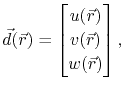(4.54)

where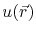,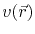, and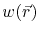are the displacements in the x, y, and z direction, respectively. The displacement is discretized on a tetrahedral element as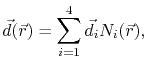(4.55)

which leads to the components discretization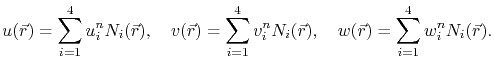(4.56)

Applying this discretization in the strain-displacement relationship (3.79), the components of the strain tensor can be written as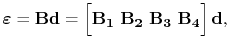(4.57)

where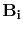is the matrix of the derivatives of the shape functions for the node i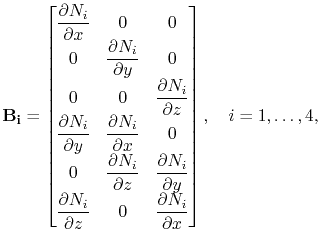(4.58)

and the displacement matrix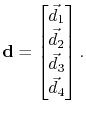(4.59)

Using (4.57), the stress-strain equation (3.81) can written as a function of the displacements according to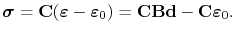(4.60)

Applying the principle of virtual work, the work of internal stresses on a continuous elastic body is given by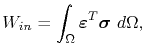(4.61)

where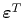is the transposed strain tensor. Combining (4.57), (4.60), and (4.61) the work on a finite element is written as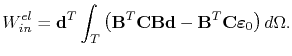(4.62)

From energy balance the internal work should be equal to the work done by external forces, i.e.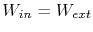, and, since during electromigration there are no external forces (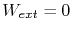), one obtains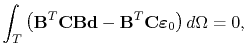(4.63)

or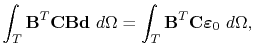(4.64)

which can be conveniently expressed as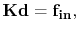(4.65)

where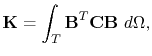(4.66)

is the so-called stiffness matrix, and(4.67)

is the internal force vector.

Equation (4.65) forms a linear system of equations of 12 equations with 12 unknowns (the three displacement components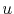,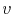, and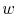for each tetrahedron node). The inelastic strain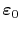determines the internal force vector according to the electromigration induced strain given by (3.78).Next: 4.3 Simulation in FEDOS Up: 4.2 Discretization of the Previous: 4.2.3 Discretization of the

R. L. de Orio: Electromigration Modeling and Simulation